Courses

# Current Electricity MSQ

## 10 Questions MCQ Test Topic wise Tests for IIT JAM Physics | Current Electricity MSQ

Description
This mock test of Current Electricity MSQ for Physics helps you for every Physics entrance exam. This contains 10 Multiple Choice Questions for Physics Current Electricity MSQ (mcq) to study with solutions a complete question bank. The solved questions answers in this Current Electricity MSQ quiz give you a good mix of easy questions and tough questions. Physics students definitely take this Current Electricity MSQ exercise for a better result in the exam. You can find other Current Electricity MSQ extra questions, long questions & short questions for Physics on EduRev as well by searching above.
*Multiple options can be correct
QUESTION: 1

### A battery of internal resistance 2Ω is connected to a variable resistor whose value can vary from 4Ω to 10Ω. The resistance is initially set at 4Ω. If the resistance is now increased then, which of the statement is not correct.

Solution:

Power maximum when r = R.
So, power consumed by it will decrease. for R > r.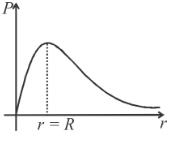The correct answers are: power consumed by it will increase, power consumed by it may increase or may decrease, power consumed will first increase then decrease.

*Multiple options can be correct
QUESTION: 2

### In the figure a conductor of non-uniform cross-section is shown. A steady current I flows in it.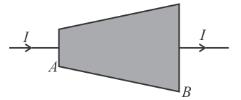Solution:

The area of cross-section of conductor at point   is less than that at point B. So current density at  A  is higher. Hence, the electric field at  A  is more than at   and the thermal power generated at  A  is more than at  B  in an element of small same width, since resistance at A  is greater.

The correct answers are: The electric field at A  is more than at  B., The thermal power generated at  A  is more than at  B  in an element of small width.

*Multiple options can be correct
QUESTION: 3

### Two cells of unequal emfs E1 and  E2 and internal resistances r1 and r2 are joined as shown in figure. VP  and  VQ  are the potential at  P and Q respectively.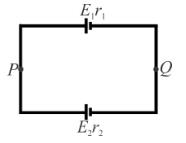Solution:

(A)  Potential difference across each cell = VP – VQ

(B)  If i is clockwise then  E2  is source and for anti-clockwise current  E1  is source.

(C)  Potential difference = E – ir (when battery supplies energy) = E + ir  (when battery consumes energy).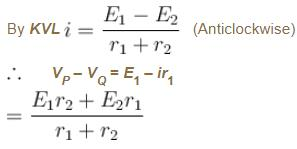The correct answers are: The potential difference across both the cell will be equal, One of the cell, will supply energy to the other cell., The potential difference across one of the cells will be greater than its emf.,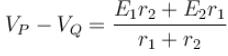*Multiple options can be correct
QUESTION: 4

In the figure shown: (All batteries are ideal)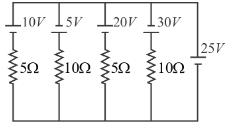Solution: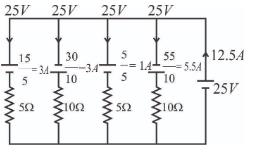Power supplied by 20V cell = (–1)(20) = – 20W
as the cell is not supplying the power, it is eating the power (getting charged)

The correct answers are: current through 25V cell is 12.5A, power supplied by 20V cell is –20W

*Multiple options can be correct
QUESTION: 5

A conductor of truncated conical (frustum) is connected to a battery of emf ∈ as shown in the figure.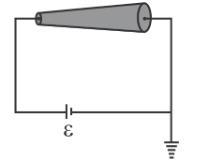If at a section distance  from left end, electric field intensity, potential and rate of generation of heat per unit length are EV  and  respectively, then which of the following graphs(s) is/are correct?

Solution:

Let  be the radius of left end side cross-section, then radius of cross-section at distance x  from left end is a + bx  where  b  is a constant.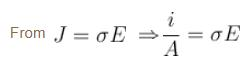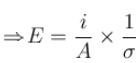as i and σ are same for all cross-section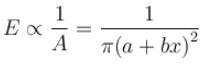Rate of heat generation per unit length,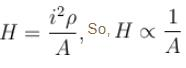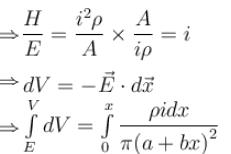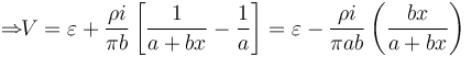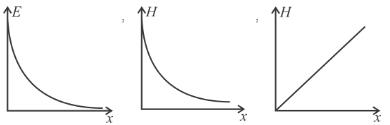*Multiple options can be correct
QUESTION: 6

In the circuit shown in the figure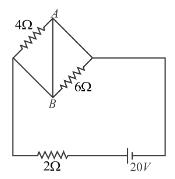Solution: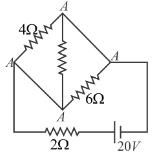there is zero potential difference across 4Ω and 6Ω resistance.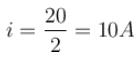power by battery Pb =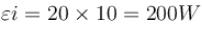The correct answers are: power supplied by the battery is 200 watt, potential difference across 4Ω resistance is equal to the potential difference across 6Ω resistance

*Multiple options can be correct
QUESTION: 7

A variable current flows through a 1Ω resistor for 2 seconds. Time dependence of the current is shown in the graph.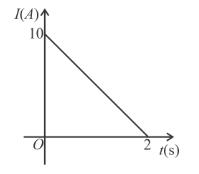Solution:

Total charge =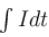= Area under the curve = 10C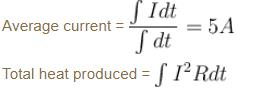Maximum Power = I2R  when I  is maximum current = 100 × 1 = 100W

The correct answers are: Total charge flown through the resistor is 10C., Average current through the resistor is 5A., Maximum power during the flow of current is 100W.

*Multiple options can be correct
QUESTION: 8

Consider a resistor of uniform cross sectional area connected to a battery of zero internal resistance. If the length of the resistor is doubled by stretching it then :

Solution:

As the length is doubled, the cross section are of the wire becomes half, thus the resistance of the wire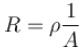becomes four times the previous value. Hence after the wire is elongated the current becomes one fourth. Electric field is potential difference per unit length and hence becomes half the initial value. The power delivered to resistance is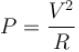and hence becomes one fourth.
The correct answers are: the electric field in the wire will become half., the thermal power produced by the resistor will become one fourth.

*Multiple options can be correct
QUESTION: 9

AB is part of a circuit as shown, that absorbs energy at a rate of 50WE is an emf device that has no internal resistance.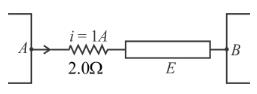Solution:

Resistance absorbs energy at the rate of 2W.

Potential difference across ABVAB·I = 50W

VAB = 50V

Drop across resistor is 2V, therefore EMF of E is 48V.

As AB is absorbing energy at the rate of 50W, 48W  is being absorbed by  E. Thus E  is on charging mode i.e. current is entering from +ve terminal of  E.

The correct answers are: Emf of the device is 48V., Rate of conversion from electrical to chemical energy is 48 in device  E.

*Multiple options can be correct
QUESTION: 10

The galvanometer shown in the figure has resistance 10Ω. It is shunted by a series combination of a resistance S = 1Ω and an ideal cell of emf 2V. A current 2A passes as shown.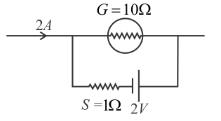Solution:

Let the currents be as shown in the figure
KVL along ABCDA
⇒  –10i – 2 + (2 – i)1 = 0
∴  i = 0
Potential difference across S = (2 – i)1 = 2 × 1 = 2V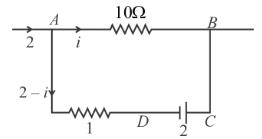The correct answers are: The reading of the galvnometer is zero, The potential difference across the resistance S  is 2V